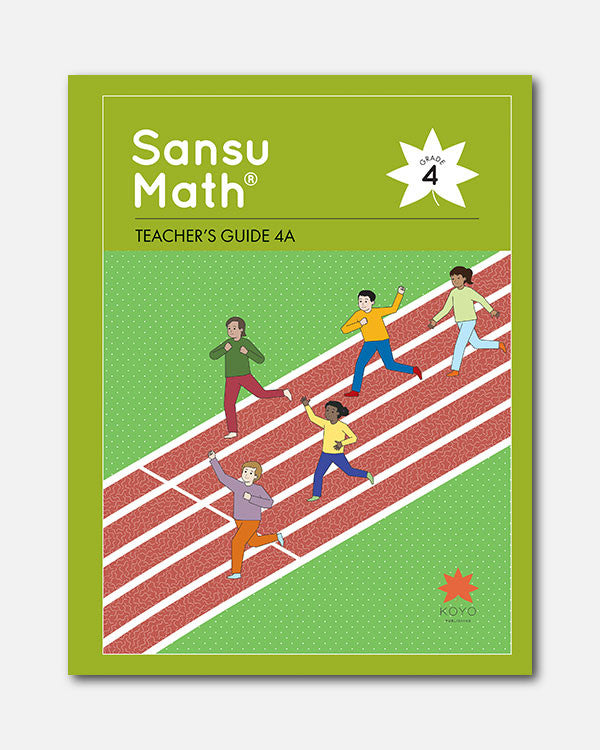# Sansu Math® Teacher's Guide 4A

In Stock
\$ 35.00

### Overview

Each unit of the Teacher's Guide begins with a unit overview that provides a discussion of core mathematical ideas, explanation of the learning trajectory for the unit and for the topic (across units), identification of key points, and ways to support students having difficulties with the unit.

The Teacher's Guides include full-color images of each page of the student textbook with overlaid answers, mirrored by a teacher’s page with lesson notes. These provide specific pedagogical suggestions, including hatsumon (key questions or problems to pose to students), anticipated responses, strategies to support student thinking, and assessment perspectives.

Lesson plans are complemented by carefully-crafted instructional boards which guide students through the units. The Teacher's Guides place an emphasis on recognizing the student's experience, incorporating varied perspectives from student characters and encouraging young learners to make discoveries on their own.

Teacher's Guides are spiral bound books designed to lay flat, and include examples of blackboard organization that can be projected using classroom technology.

Sansu Math® Teacher's Guide 4A is written for use with Sansu Math® Grade 4 student textbook for units 1-7.

1. Let's Investigate Numbers Greater Than a Hundred Million
1. Structure of Large Numbers
2. Structure of Whole Numbers
3. Multiplication
2. Let's Investigate How to Express the Size of Angles
3. Let's Think about How to Calculate Division
1. Dividing Multiples of 10 and 100
2. Division Algorithm (1)
3. Division Algorithm (2)
4. Calculations with Times as Much
5. Mental Calculation
4. Let's Make Quadrilaterals
1. Intersecting Lines
2. Arrangements of Lines
5. Let's Represent How Quantities Change in Graphs
6. Let's Investigate the Structure of Decimal Numbers
1. How to Express Decimal Numbers
2. Structure of Decimal Numbers
3. Addition and Subtraction of Decimal Numbers
7. Let's Think about the Division Algorithm
1. Dividing by Multiples of 10
2. Division Algorithm with 2-digit divisors (1)
3. Division Algorithm with 2-digit divisors (2)
4. Properties of Division